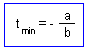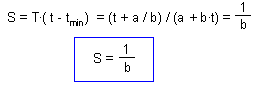## 8.2. Simple degree-day model

Degree-day model is based on the assumption that development rate is a linear function of temperature.

In most cases, real development rate is indeed a linear function in the region of moderate temperatures (15-25°) (see figure above). Deviating points at temperatures that are too low or too high can be ignored. For example, in the figure, regression line is plotted for points from 10 to 30°. At 5° all organisms died and there was no development. At 35° organisms were overheated and development rate of survivors was reduced. If we are interested in simulating organism development in moderate temperatures, then the degree-day model will be the best choice.

Terms:

• tmin is the lower temperature limit; this is the temperature at which development rate reaches zero.
• ET = t - tmin is effective temperature.
• S are degree-days; this is the effective temperature multiplied by the number of days required to complete development.
Lower temperature limit and degree-days can be estimated from the regression line of development rate versus temperature. We assume that regression equation is:

v = a + bt

where a is intercept, and b slope.

a + btmin = 0, by the definition of the lower temperature limit. Thus,In our case, tmin = 0.0104 / 0.00142 = 7.3°.

Duration of development is T = 1/v. Thus, degree-days can be estimated as:This equation shows that degree-days do not depend on temperature! This is the principal feature of the degree-day model. In our case, S = 1 / 0.00142 = 704 degree × day. The units of degree-days are degrees (centigrade) multiplied by days. Some times, it is better to use degree-hours if development is very fast.

Because degree-days are temperature-independent, it is possible to use them to predict development time in experiments with variable temperature. In this case effective temperatures are accumulated day by day, and when the sum reaches S, then development is finished.

Example: tmin = 10 , S = 100.

 Day No. AverageTemperature t Effective temperature, t - tmin Accumulateddegree-days 1 15 5 5 2 18 8 13 3 25 15 28 4 23 13 41 5 24 14 55 6 18 8 63 7 17 7 70 8 15 5 75 9 18 8 83 10 15 5 88 11 22 12 100 12 25 15 115

Accumulated degree-days reach the value S = 100 on day 11. Thus, it takes 11 days to complete the development.

Alexei Sharov 12/5/98